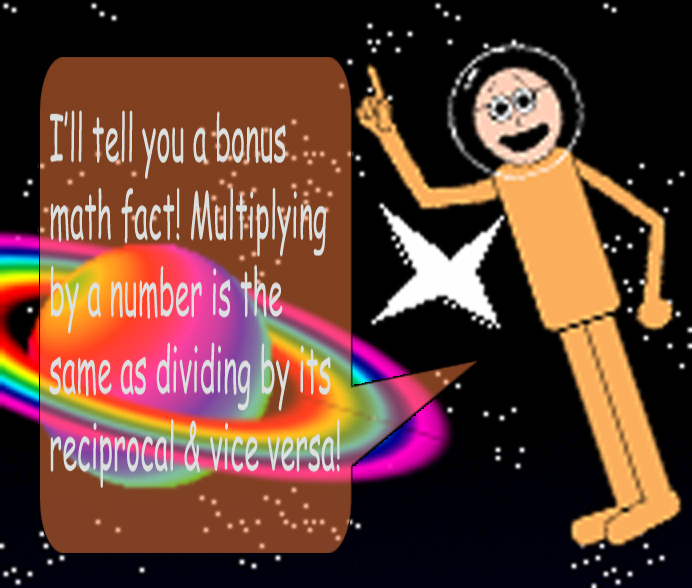# Magic Cut

## Examples:

x = 100, y = 4; x^2 = 10,000

10,000 ÷ 4 = 2,500

2,500/100 = 2,500 ÷ 100 = 25

100/4 = 100 ÷ 4 = 25

The number 25 may not be a fraction, but all integers can be printed as fractions (non-algebraic ones) since they're all rational! Plus, some fractions are equal to the same number!

## 2 = 2/1 = 4/2 = 6/3 = 8/4 = 10/5 = 12/6 =14/7 = 16/8 = 18/9 = 20/10 = etc.

As long as the numerator is twice as big as the denominator, the fraction/quotient is equal to 2. Don't forget it!

x = -18, y = 3; x^2 = 324

324 ÷ 3 = 108

108/-18 = 108 ÷ -18 = -6

-18/3 = -18 ÷ 3 = -6

Both division problems & fractions gave us the same quotient!

Even if the variables are negative, this trick still works! It'll even work with non-integers!

x = 12, y = ¼; x^2 = 144

144 ÷ ¼ = 144 × 4 = 576

576/12 = 576 ÷ 12 = 48

12/(¼) = 12 × 4 = 48x = i, y = 1/5; x^2 = -1

-1 ÷ 1/5 = -1 × 5 = -5

-5/i = -5 × -i = 5i

5i = x/y

...And imaginary/complex numbers! (Remember that negative real numbers have imaginary square roots.)